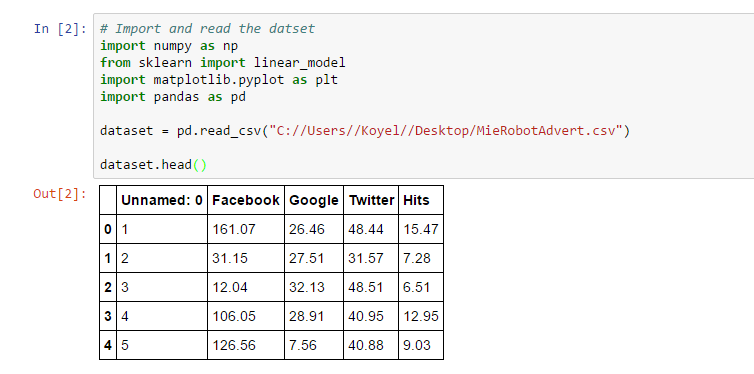# Easy Linear Regression for anyone in 10 stepsLinear regression is the first machine learning algorithm which we will blog along with Python code today.

What we plan to achieve in this blog?

We will use the Scikit learn package to train the algorithm over a dataset which we have provided from MieRobot advert set. We would also see if there is a linear relation between 'Hits/Likes' to the multivariate variables of per day cost of the advert in facebook, google and twitter. This is a dummy dataset which we have neutralised. It may not make total sense but the intention is to have a running learning algorithm which anyone can use.You may change the dataset as you wish and try other dataset sources which we will provide the link for.

Linear Regression mathematics you need to know

The mathematics of linear regression is beyond the scope of this blog. Please note theories are very important and you would need to know the below concepts of mathematics.

• Cost Function

• Contour lines

• Aware of metrics as mean square error

The prediction model of linear regression takes the equation as:

min (a,b) [H(x) - y] ^2

Where,

• y is the value we know from supervised learning

• H(x) is the actual predictions we make from our linear model

• a,b are the parameters for which we need to find the minimum

What are the prerequisites?

You would need to have a laptop with at least 4 GB RAM and have Python installed preferably using the anaconda distribution of version 3.x. Get seaborn, Jupyter notebook installed along with matplotlib as well.

Anaconda blog from MieRobot

So lets code in Python now.

We are using Jupyter Notebook which you can download here. You can also download a single Python program. (If you want the code directly scroll down at the end of the blog to download section).

Step 1: We import the needed libraries and we will use the Pandas dataset for this blog as it is very handy for data visualisation. As we will plot within the Jupyter notebook we also make the inline call in this step. If you are using Anaconda please conda install the needed packages. Else PIP install the missing packages.Step 2: (Optional) We do some basic checks on the dataset by using the columns and describe options from Pandas.Step 3: (Optional) We create a seaborn pair plot and heat map which comes in very handy to visualise the data in multivariate datasets.Step 4: Now we move to the machine learning part as we have a fairly decent idea of the dataset using the visualisation methods in steps 2,3. We will use the Skikit learn of train, test and split where we use the data in 3 parts and 40% is used in the test. We stick to the normal format of the standard parameters and use a random state of 101. You can experiment by changing the test and random state as an exercise.Step 5: We now move in the linear regression part and use the scikit learn function for linear regression and fit over the dataset in the previous step.Step 6: We calculate and call the intercept, Coefficient. We then call the prediction over the dataset.Step 7: Plot the scatter diagram from the results using standard matplotlib.Step 8: Check the distribution plot of the hits predicted.Step 9: Show the metrics of the predictions using mean square error, mean absolute error and mean squared error. We see that our model is pretty decent and does a good job at prediction.Step 10: (Further work) This is just a starter approach to enable you to write your first learning algorithm on machine learning. As part of further work you may -

• You may play around with other datasets from various sources and see if you can derive a linear regression.

• You can also change the test size and compare the results.

• If you look carefully at the dataset, there the first column (unnamed) can be dropped. You can drop this column before train-test-split and see your results.

Sources of dataset which you may use for further work

There are many sources of dataset which you may use for your learning algorithm. We have captured some for your references as:

• World Bank dataset: World bank provides data and also visualisation

• Data.Gov: Home of open data from USA Government

• Data India: Home of Open data from Government of India. Files are offered to be downloaded in many formats and data is from wide areas

• UCI machine learning repository: This is very good site for machine learning dataset and what we like here is that it tells you if a particular dataset is suited for classification, regression etc

• MNIST dataset: This is for image recognition for hand written digits.We plan to blog on this dataset for our Opencv and computer vision section soon.